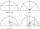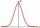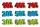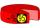The square

The square root of 25 times the square root of 81 is what number?

Result

x =  45

Solution:Leave us a comment of this math problem and its solution (i.e. if it is still somewhat unclear...):Be the first to comment!Next similar math problems:

1. Numeric questionIt is possible negative irrational number?
2. AverageAverage of 7 numbers is 65. What is its sum?
3. Double percentWhat is 80% of 60% of 2800?
4. Watching TVOne evening 2/3 students watch TV. Of those students, 3/8 watched a reality show. Of the students that watched the show, 1/4 of them recorded it. What fraction of the students watched and recorded reality tv.
5. New refrigeratorNew refrigerator sells for 1024 USD, Monday will be 25% discount. How much USD will save, and what will be the price?
6. GlovesI have a box with two hundred pieces of gloves in total, split into ten parcels of twenty pieces, and I sell three parcels. What percent of the total amount I sold?
7. Unknown numberIdentify unknown number which 1/5 is 40 greater than one tenth of that number.
8. Conference148 is the total number of employees. The conference was attended by 22 employees. How much is it in percent?
9. PercentagesExpressed as a percentage:
10. MO 2016 Numerical axisCat's school use a special numerical axis. The distance between the numbers 1 and 2 is 1 cm, the distance between the numbers 2 and 3 is 3 cm, between the numbers 3 and 4 is 5 cm and so on, the distance between the next pair of natural numbers is always in
11. InequationSolve the inequation: 5k - (7k - 1)≤ 2/5 . (5-k)-2
12. Apples 2James has 13 apples. He has 30 percent more apples than Sam. How many apples has Sam?
13. Base, percents, valueBase is 344084 which is 100 %. How many percent is 384177?
14. Sales offGoods is worth € 70 and the price of goods fell two weeks in a row by 10%. How many % decreased overall?
15. Percents - easyHow many percent is 432 out of 434?
16. Simplify 2Simplify expression: 5ab-7+3ba-9
17. TVsProduction of television sets increased from 3,500 units to 4,200 units. Calculate the percentage of production increase.